# 5G NR Downlink CSI Reporting

This example shows how to compute downlink channel state information (CSI) parameters such as the channel quality indicator (CQI), precoding matrix indicator (PMI), and rank indicator (RI), for multiple input multiple output (MIMO) scenarios, as defined in TS 38.214 Section 5.2.2, over a tapped delay line (TDL) channel. The example supports CSI parameter computation for the type I single-panel, type I multi-panel, type II codebooks, and enhanced type II codebooks.

### Introduction

CSI parameters are the quantities related to the state of a channel. The user equipment (UE) reports CSI parameters to the access network node (gNB) as feedback. The CSI feedback includes several parameters, such as the CQI, the PMI, and the RI. The UE uses the channel state information reference signal (CSI-RS) to measure the CSI feedback. Upon receiving the CSI parameters, the gNB schedules downlink data transmissions (such as modulation scheme, code rate, number of transmission layers, and MIMO precoding) accordingly. This figure shows an overview of CSI-RS transmission, CSI computation and feedback, and the transmission of downlink data that is scheduled based on the CSI parameters.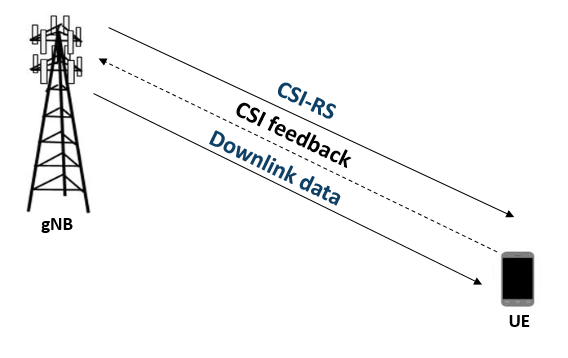#### RI Selection

The RI defines the number of possible layers for the downlink transmission under specific channel conditions. The RI also corresponds to the maximum number of uncorrelated paths that the downlink transmission can use. Other CSI parameters like the PMI and CQI are computed based on the rank provided by the RI. For given channel conditions, the `hRISelect` function computes the CQI values for all valid values of number of transmission layers. The function returns the number of transmission layers that have maximum modulation and coding efficiency.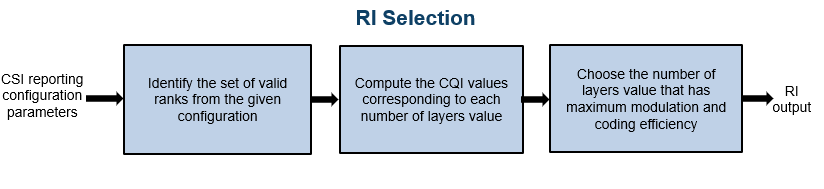#### PMI Selection

The PMI consists of a set of indices corresponding to the precoding matrix. The gNB can apply this precoding matrix for the downlink data transmission. The `hDLPMISelect` function reports a precoding matrix by considering a codebook from these supported codebooks:

• Type I single-panel codebooks, as defined in TS 38.214 Tables 5.2.2.2.1-1 to 5.2.2.2.1-12

• Type I multi-panel codebooks, as defined in TS 38.214 Tables 5.2.2.2.2-1 to 5.2.2.2.2-6

• Type II codebooks, as defined in TS 38.214 Tables 5.2.2.2.2-1 to 5.2.2.2.3-5

• Enhanced type II codebooks, as defined in TS 38.214 Tables 5.2.2.2.5-1 to 5.2.2.2.5-6

The PMI selection is based on the codebook type, the number of transmission layers, and other CSI reporting configuration parameters such as antenna panel dimensions. Each codebook consists of a set of precoding matrices. NR considers a dual-stage precoding matrix based on the codebooks design from TS 38.214 Sections 5.2.2.2.1 to 5.2.2.2.3 and Section 5.2.2.2.5.

`$\mathit{W}={\mathit{W}}_{1}{\mathit{W}}_{2}$`

Where,

${\mathit{W}}_{1}$ is a matrix representing a discrete Fourier transform (DFT) beam or beam group for both polarizations based on the codebook type

${\mathit{W}}_{2}$ can be any of the following based on the codebook type:

• Beam selection from ${\mathit{W}}_{1}$

• Weighting coefficients for the beams in ${\mathit{W}}_{1}$

• Cophasing values between two polarizations

For type I single-panel and multi-panel codebooks, the function computes signal to interference plus noise ratio (SINR) at the receiver side for all precoding matrices from the selected codebook and for the given channel conditions. The function reports the PMI as the set of indices ${\mathit{i}}_{1}$, ${\mathit{i}}_{2}$, as defined in TS 38.214 Section 5.2.2.2.1 for type I single-panel codebooks and TS 38.214 Section 5.2.2.2.2 for type I multi-panel codebooks. These indices correspond to a precoding matrix $\mathit{W}$, which gives the maximum SINR. Type I single and multi-panel codebooks are useful for single-user MIMO scenarios. This figure shows the procedure for PMI selection from type I single-panel and multi-panel codebooks.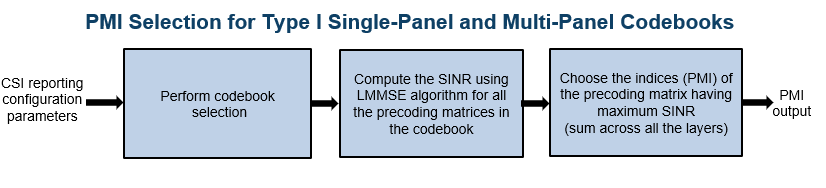Type II codebooks consider a set of orthogonal DFT beams to form the precoding matrix. As mentioned above, the ${\mathit{W}}_{1}$ matrix contains the information related to the DFT beams. For the given channel conditions and the number of DFT beams, the function computes the beam amplitude scaling and cophasing values (${\mathit{W}}_{2}$) for all the DFT beams in each orthogonal beam group so that the linear combination of orthogonal beams approximates the eigenvector of the channel. The function reports the indices ${\mathit{i}}_{1}$and ${\mathit{i}}_{2}$, as defined in TS 38.214 Section 5.2.2.2.3. These indices correspond to the precoding matrix $\mathit{W}$, which gives the maximum SINR. Type II codebook based PMI is more accurate than type I codebook based PMI, as multiple beams are considered in type II codebooks for the approximation of channel eigenvector. Because type II codebooks consider multiple beams, multi-user MIMO scenarios use type II codebooks instead of type I codebooks. These figures show the PMI selection procedure from type II codebooks.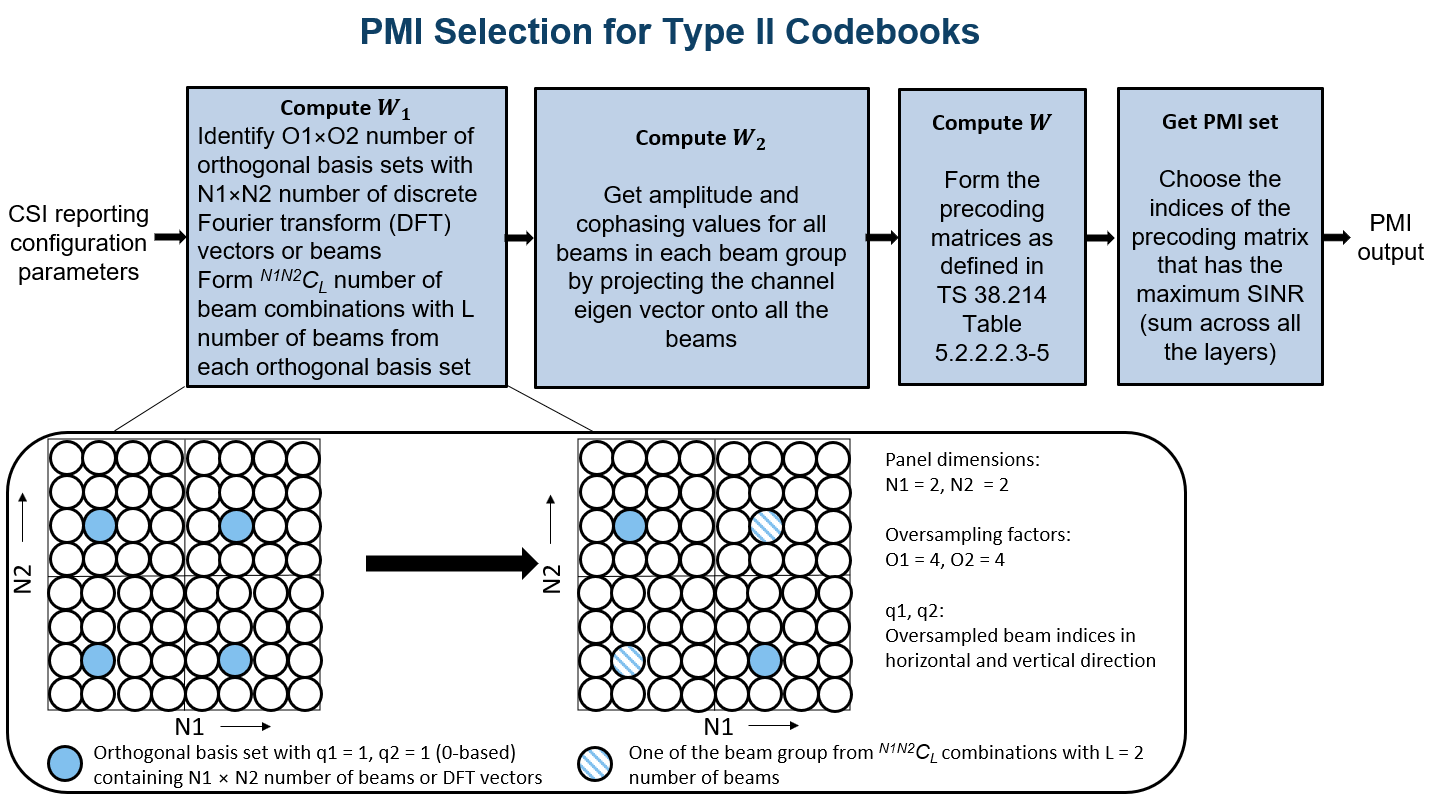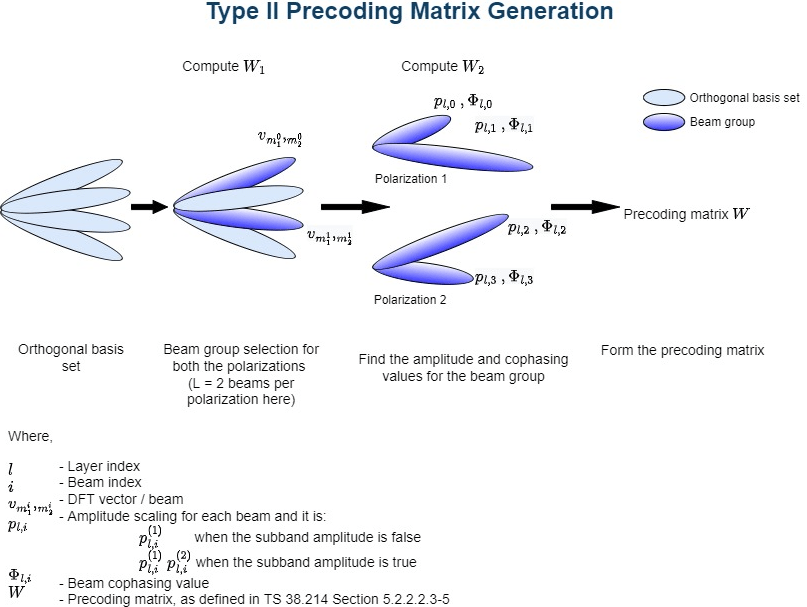This figure shows the quantization process in space domain (i.e., few beams in the approximation of channel eigenvector) in type II codebooks to form ${\mathit{W}}_{2}$ matrix for each layer.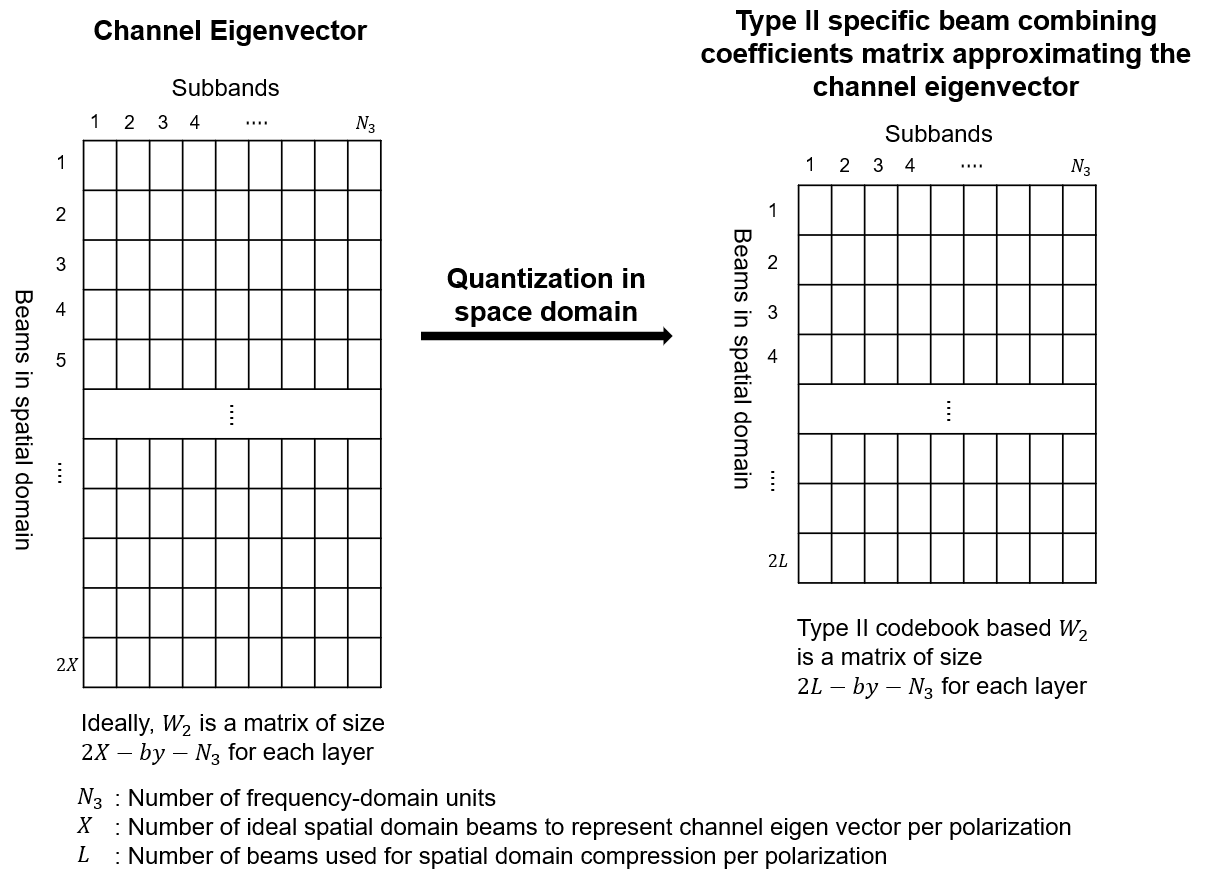Because type II codebooks support only up to rank 2, the standard introduces enhanced type II codebooks. The PMI computation that is based on enhanced type II codebooks extends type II codebooks by supporting up to rank 4 with one overhead reduction technique. The beam amplitude scaling and cophasing values (also called beam combining coefficients) for all the beams are computed similar to type II codebooks approach. With the rank extension, the feedback overhead increases linearly and with the number of subbands. For this amount of feedback, the uplink resources may not be sufficient. By taking the advantage of the frequency-domain correlation of beam combining coefficients, a process called DFT or frequency-domain compression is employed for enhanced type II codebooks to reduce the feedback overhead.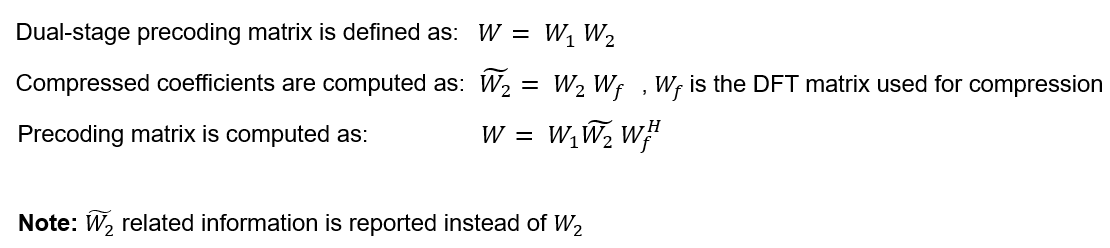The figure shows the frequency-domain compression process.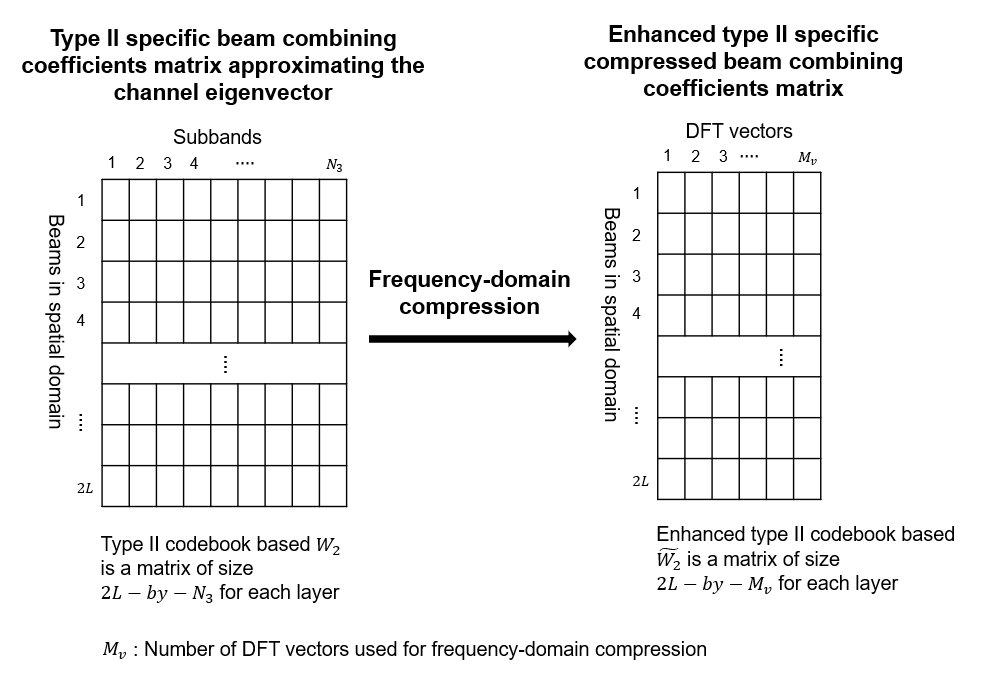Along with frequency-domain compression, enhanced type II codebooks limit the number of nonzero coefficients in the feedback by ignoring the weaker beam combining coefficients.

#### CQI Selection

The CQI is an indicator of channel quality. The CQI value is a scalar in the range [0, 15]. The CQI value provides information about the highest modulation scheme and the code rate (MCS) suitable for the downlink transmission to achieve the required block error rate (BLER) for given channel conditions.

The CSI reference resource is a group of downlink frequency-domain and time-domain resources that are configured as defined in TS 38.214 Section 5.2.2.5. The gNB transmits a single physical downlink shared channel (PDSCH) transport block occupying the resource blocks called the CSI reference resource, with a combination of modulation scheme and target code rate that correspond to each CQI index. The UE selects the highest possible CQI, when the PDSCH transport block can be received with a transport block error probability not exceeding:

• 0.1 when 'cqi-Table' is 'table1' or 'table2'

• 0.00001 when 'cqi-Table' is 'table3'

The 'cqi-Table' is a higher layer parameter that corresponds to the CQI versus MCS table, and the SINR lookup table is computed for this table. This example uses 'cqi-Table' as 'table1' (TS 38.214 Table 5.2.2.1-2). The relationship between the CQI indices, the modulation scheme, and the code rate (from which the transport block size is derived) is described in TS 38.214 Tables 5.2.2.1-2 to 5.2.2.1-4.

The `hCQISelect` function computes the CQI value by considering the SINR values that correspond to the reported PMI. This function uses the precalculated lookup table of the CQI index versus ${\mathrm{SINR}}_{\mathit{i}}$, as a reference, if you provide it.

#### CQI Selection Using SINR Lookup Table

The function maps the SINR values across all layers (corresponding to the reported PMI) to each codeword. For each codeword, the function compares the respective SINR with the ${\mathrm{SINR}}_{\mathit{i}}$ values from the table and then selects the CQI value against the maximum SINR, which is less than the codeword SINR. The function sets the CQI value so that the BLER is less than or equal to 0.1 when operated at ${\mathrm{SINR}}_{\mathit{i}}$. If a CQI index of 1 does not satisfy the BLER condition, then the function sets the CQI index to 0. This figure shows the procedure for CQI selection.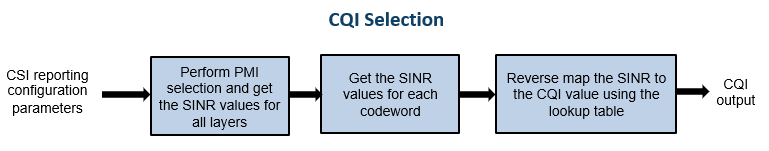#### CQI Selection Without SINR Lookup Table

The function computes the CQI values by using the SINR values across all layers corresponding to the reported PMI. Based on the number of codewords, the function forms the combinations of CQI values and reports the one which satisfies the BLER condition.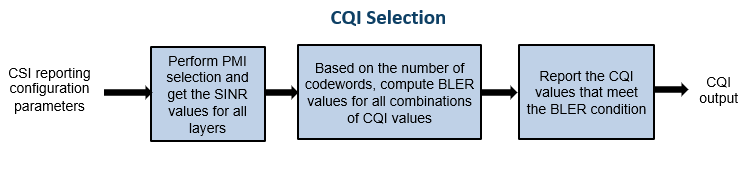#### CQI Versus SINR Table

To create the lookup tables, update the NR PDSCH Throughput example by configuring the CSI reference resource, as defined in TS 38.214 Section 5.2.2.5. Perform simulations for PDSCH throughput by specifying the modulation scheme and code rate corresponding to each CQI, the channel conditions, and a finite range of SINR values with zero interference. Map the SINR value observed at the receiver against each CQI index when the target BLER is achieved. This figure shows the procedure for CQI versus SINR table creation.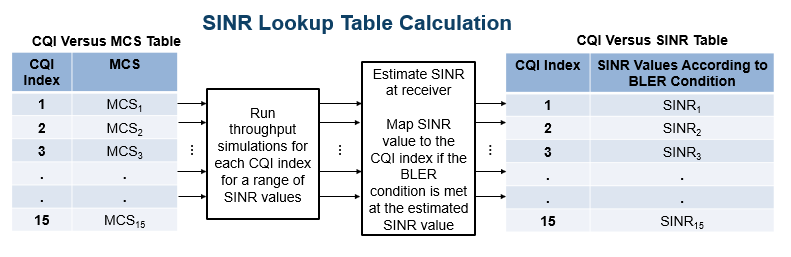#### Outline of Example

This example shows how to compute the CQI and PMI indices for a 4-by-4 MIMO scenario over a TDL channel with the TDL-C delay profile, a delay spread of 300 nanoseconds, and a maximum Doppler shift of 50 Hz. It compares the time-domain and frequency-domain variations of CQI values against the time-domain and frequency-domain variations of SINR values for practical and perfect channel estimation scenarios. The example also highlights the time-domain and frequency-domain variations of the PMI values corresponding to the reported rank for practical and perfect channel estimation scenarios.

### Simulation Length and SNR Point

Set the length of the simulation in terms of the number of 10 ms frames. The SNR (SINR with zero interference) is defined per resource element (RE) and applies to each receive antenna. For an explanation of the SNR definition that this example uses, see SNR Definition Used in Link Simulations.

```nFrames = 5; % Number of 10 ms frames SNRdB = 10; % SNR in dB```

### Carrier, Bandwidth Part, and CSI-RS Configuration

Create a carrier configuration object representing a 10 MHz carrier with a subcarrier spacing of 15 kHz.

```carrier = nrCarrierConfig; carrier.SubcarrierSpacing = 15; carrier.NSizeGrid = 52;```

Configure the size of the bandwidth part (BWP) and the start of the BWP relative to common resource block 0 (CRB 0).

```NStartBWP = 0; NSizeBWP = 52;```

Create a CSI-RS configuration object representing a non-zero-power CSI-RS (NZP-CSI-RS) resource set with a set of NZP-CSI-RS resources. Make sure that the NZP-CSI-RS resources have the same code division multiplexing (CDM) types and the same number of CSI-RS ports, as defined in TS 38.214 Section 5.2.2.3.1.

```csirs = nrCSIRSConfig; csirs.CSIRSType = {'nzp','nzp','nzp'}; csirs.RowNumber = [4 4 4]; csirs.NumRB = 52; csirs.RBOffset = 0; csirs.CSIRSPeriod = [4 0]; csirs.SymbolLocations = {0, 0, 0}; csirs.SubcarrierLocations = {0, 4, 8}; csirs.Density = {'one','one','one'};```

Configure the number of transmit and receive antennas. The number of transmit antennas must be equal to the number of CSI-RS ports because this example does not consider the mapping of antenna ports to physical antennas.

```nTxAnts = csirs.NumCSIRSPorts(1); nRxAnts = 4;```

Validate the CSI-RS configuration object.

`validateCSIRSConfig(carrier,csirs,nTxAnts);`

### CSI Reporting Configuration

Specify the parameters for the CQI, PMI, and RI computation as a structure with these fields:

• `NSizeBWP` — Size of the BWP in terms of the number of physical resource blocks (PRBs)

• `NStartBWP` — Starting PRB index of the BWP relative to CRB 0

• `CQITable` — Channel quality indicator table (`'table1'`, `'table2'`, `'table3'`), as defined in TS 38.214 Tables 5.2.2.1-2 to 5.2.2.1-4

• `CodebookType` — Codebook type for CSI parameter computation (`'Type1SinglePanel'`, `'Type1MultiPanel'`, `'Type2'`, `'eType2'`)

• `PanelDimensions` — Antenna panel dimensions corresponding to the `CodebookType` field

If `CodebookType` is set to any one of (`'Type1SinglePanel'`, `'Type2'`, `'eType2'`), the panel dimensions are in the form of [N1 N2] according to TS 38.214 Table 5.2.2.2.1-2. This figure shows the supported panel dimensions.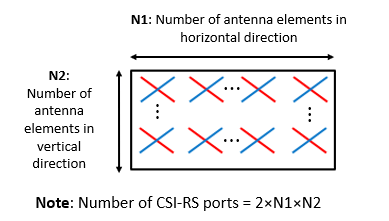If `CodebookType` is set to '`Type1MultiPanel'`, the panel dimensions are in the form of [Ng N1 N2] according to TS 38.214 Table 5.2.2.2.2-1. This figure shows the supported type I multi-panel dimensions.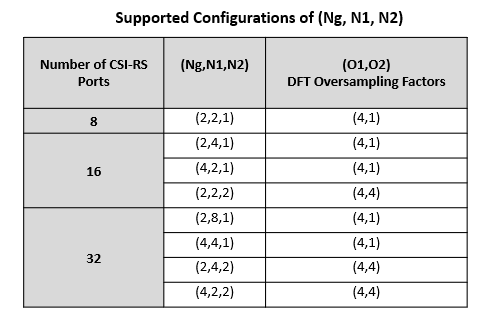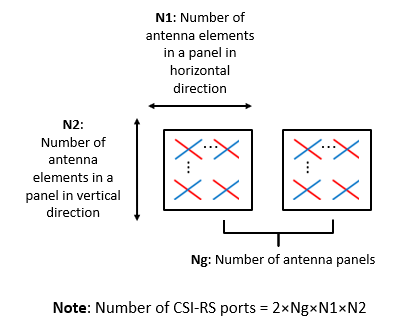• `CQIMode` — Mode of CQI reporting (`'Subband'`, `'Wideband'`)

• `PMIMode` — Mode of PMI reporting (`'Subband'`, `'Wideband'`)

• `SubbandSize` — Size of the subband, as defined in TS 38.214 Table 5.2.1.4-2 (required only when `CQIMode` or `PMIMode` is `'Subband'`)

• `PRGSize` — Precoding resource block group (PRG) size for the CQI calculation (required only when the reporting must be done for the report quantity 'cri-RI-i1-CQI' and `CodebookType` is configured as `'Type1SinglePanel'`, as defined in TS 38.214 Section 5.2.1.4.2)

• `CodebookMode` — Codebook mode according to which the codebooks are derived. The value must be 1 or 2. This field is applicable only when the `CodebookType` is specified as `'Type1SinglePanel'` or `'Type1MultiplePanel'. `When the `CodebookType` is specified as `'Type1SinglePanel'`, this field is required only when the number of transmission layers is 1 or 2 and the number of CSI-RS ports is greater than 2. When the `CodebookType` is specified as `'Type1MultiplePanel'`, this field is required for all the number of transmission layers, but the `CodebookMode` value 2 is applicable only for the panel configurations with Ng value 2.

• `CodebookSubsetRestriction` — For type I single-panel and multi-panel codebooks, it is a restriction parameter pertaining to the codebook index set ${\mathit{i}}_{1}$, that is, ${\mathit{v}}_{\mathrm{lm}}$ or ${\stackrel{~}{\mathit{v}}}_{\mathrm{lm}}$ restriction parameter, as defined in TS 38.214 Section 5.2.2.2.1. This field denotes the set of ${\mathit{i}}_{1}$ indices ([${\mathit{i}}_{11\text{\hspace{0.17em}}}$, ${\mathit{i}}_{12\text{\hspace{0.17em}}}$]) that are restricted from the consideration for the PMI computation. For type II codebooks, this field restricts the maximum amplitudes that are allowed for four vector groups indicated by ${\mathit{r}}_{1}^{\left(\mathit{k}\right)},\text{\hspace{0.17em}}{\mathit{r}}_{2}^{\left(\mathit{k}\right)}$ for $\mathit{k}=0,1,2,3$, as defined in TS 38.214 Section 5.2.2.2.3. For enhanced type II codebooks, this field restricts the maximum average amplitude coefficients that are allowed for four vector groups indicated by ${\mathit{r}}_{1}^{\left(\mathit{k}\right)},\text{\hspace{0.17em}}{\mathit{r}}_{2}^{\left(\mathit{k}\right)}$ for $\mathit{k}=0,1,2,3$, as defined in TS 38.214 Section 5.2.2.2.5. In any of the codebook types, this field is specified as a bitmap.

• `i2Restriction` — Restriction parameter pertaining to the codebook index ${\mathit{i}}_{2}$. This field denotes the set of ${\mathit{i}}_{2}$ indices that are restricted from the consideration for the PMI computation. This field is applicable only when `CodebookType` is `'Type1SinglePanel'`.

• `RIRestriction` — Restriction parameter pertaining to the rank indicator, which implies the set of ranks that are restricted from usage for the RI computation.

• `NumberOfBeams` — Number of beams in the beam group per polarization. This field is applicable only when the `CodebookType` is specified as `'Type2'`. The value must be one of {2, 3, 4}.

• `PhaseAlphabetSize` — Represents the range of the phases that are considered for the computation of ${\mathit{i}}_{2}$ indices. This field is applicable only when the `CodebookType` is specified as `'Type2'`. The value must be one of {4, 8}. The value 4 represents the phases corresponding to QPSK and the value 8 represents the phases corresponding to 8-PSK.

• `SubbandAmplitude` — Logical scalar that enables the reporting of differential amplitudes per subband when set to `true` and disables differential subband amplitude in PMI reporting when set to `false`. The value must be one of {`true`, `false`}. This field is applicable when `CodebookType` is specified as `'Type2'` and `PMIMode` is `'Subband'`.

• `ParameterCombination `— It is a positive scalar integer in the range 1...8. This is applicable when `CodebookType` is specified as `'eType2'`. This parameter defines the number of beams and two other parameters as defined in TS 38.214 Table 5.2.2.2.5-1.

• `NumberOfPMISubbandsPerCQISubband` — Represents the number of PMI subbands within one CQI subband, as defined in TS 38.214 Section 5.2.2.2.5. It is a positive scalar integer, and it must be either 1 or 2.

Configure CSI reporting configuration parameters.

```reportConfig.NStartBWP = NStartBWP; reportConfig.NSizeBWP = NSizeBWP; reportConfig.CQITable = 'table1'; reportConfig.CodebookType = 'Type1SinglePanel'; reportConfig.PanelDimensions = [2 1]; reportConfig.CQIMode = 'Subband'; reportConfig.PMIMode = 'Subband'; reportConfig.SubbandSize = 4; reportConfig.PRGSize = []; reportConfig.CodebookMode = 1; reportConfig.CodebookSubsetRestriction = []; reportConfig.i2Restriction = []; reportConfig.RIRestriction = []; reportConfig.NumberOfBeams = 2; % Applicable only when CodebookType is 'Type2' reportConfig.SubbandAmplitude = false; % Applicable only when CodebookType is 'Type2' reportConfig.PhaseAlphabetSize = 4; % Applicable only when CodebookType is 'Type2' reportConfig.ParameterCombination = 2; % Applicable only when CodebookType is 'eType2' reportConfig.NumberOfPMISubbandsPerCQISubband = 2; % Applicable only when CodebookType is 'eType2'```

### Propagation Channel Model Configuration

Create a TDL channel with the TDL-C delay profile, a delay spread of 300 nanoseconds, and a maximum Doppler shift of 50 Hz.

```channel = nrTDLChannel; channel.NumTransmitAntennas = nTxAnts; channel.NumReceiveAntennas = nRxAnts; channel.DelayProfile = 'TDL-C'; channel.MaximumDopplerShift = 50; channel.DelaySpread = 300e-9;```

Set the sampling rate for the channel model by using the value that the `nrOFDMInfo` function returns.

```waveformInfo = nrOFDMInfo(carrier); channel.SampleRate = waveformInfo.SampleRate;```

Get the maximum number of delayed samples by a channel multipath component. The maximum number of delayed samples is calculated from the channel path with the largest delay and the implementation delay of the channel filter. The number of samples that correspond to the maximum channel delay is required later to flush the channel filter to obtain the received signal.

```chInfo = info(channel); maxChDelay = ceil(max(chInfo.PathDelays*channel.SampleRate)) + chInfo.ChannelFilterDelay;```

### Processing Loop

For each slot, generate the CSI-RS, transmit it through the channel, and then process at the receiver side to compute the CQI, PMI, and RI values. Follow the steps for the transmit-to-receive processing for each slot.

1. Generate the resource grid — Generate the slot grid that contains the CSI-RS and map the slot grid from CSI-RS ports to transmit antennas.

2. Generate the waveform — Perform orthogonal frequency division multiplexing (OFDM) modulation on the generated grid by using the `nrOFDMModulate` function.

3. Model and apply a noisy channel — Pass the waveform through a TDL-C fading channel, and then add the additive white Gaussian noise (AWGN). The SNR is defined per RE and applies to each receive antenna.

4. Perform timing synchronization and OFDM demodulation — For practical synchronization, correlate the received waveform with the CSI-RS. For perfect synchronization, use the path gains and path filters of the channel. Perform OFDM demodulation on the synchronized signal using the `nrOFDMDemodulate` function.

5. Perform channel estimation — For practical channel estimation, use the CSI-RS. For perfect channel estimation, use the path gains, the path filters, and the sample times of channel snapshots.

6. Compute CQI, PMI, and RI values — Compute CQI, PMI, and RI values using practical and perfect channel estimations.

Calculate total number of slots.

`totSlots = nFrames*carrier.SlotsPerFrame;`

Initialize variables to store CQI, PMI, RI, and subband SINR values for practical and perfect channel estimation scenarios.

After the completion of processing loop:

• The variables `cqiPracticalPerSlot`, `cqiPerfectPerSlot`, `SINRPerSubbandPerCWPractical`, and `SINRPerSubbandPerCWPrerfect` are multidimensional arrays of size `numSBs`-by-2-by-`totSlots`. The `numSBs` value is equal to the number of subbands plus 1, that is, the corresponding wideband value followed by subband values. The second dimension value denotes the maximum possible number of codewords.

• The variables `pmiPracticalPerSlot` and `pmiPerfectPerSlot` are arrays of structures of size `totSlots`. The structure represents the PMI values with fields `i1` and `i2`.

• The variables `riPracticalPerSlot` and `riPerfectPerSlot` are arrays of size 1-by-`totSlots`.

```cqiPracticalPerSlot = []; subbandCQIPractical = []; pmiPracticalPerSlot = struct('i1',[],'i2',[]); SINRPerSubbandPerCWPractical = []; cqiPerfectPerSlot = []; subbandCQIPerfect = []; pmiPerfectPerSlot = struct('i1',[],'i2',[]); SINRPerSubbandPerCWPerfect = []; riPracticalPerSlot = []; riPerfectPerSlot = []; % Get number of CSI-RS ports csirsPorts = csirs.NumCSIRSPorts(1); % Get CDM lengths corresponding to configured CSI-RS resources cdmLengths = getCDMLengths(csirs); % Initialize the practical timing offset as zero. It is updated in the % slots when the correlation is strong for practical synchronization. offsetPractical = 0; % Initialize a variable to store the information of the slots % where NZP-CSI-RS resources are present. It is of length totSlots. totSlotsBinaryVec = zeros(1,totSlots); % Set RNG state for repeatability rng('default'); % Loop over all slots for nslot = 0:totSlots - 1 % Create carrier resource grid for one slot csirsSlotGrid = nrResourceGrid(carrier,csirsPorts); % Update slot number in carrier configuration object carrier.NSlot = nslot; % Generate CSI-RS indices and symbols csirsInd = nrCSIRSIndices(carrier,csirs); csirsSym = nrCSIRS(carrier,csirs); % Map CSI-RS to slot grid csirsSlotGrid(csirsInd) = csirsSym; % Map CSI-RS ports to transmit antennas wtx = eye(csirsPorts,nTxAnts); txGrid = reshape(reshape(csirsSlotGrid,[],csirsPorts)*wtx,size(csirsSlotGrid,1),size(csirsSlotGrid,2),nTxAnts); % Perform OFDM modulation to generate time-domain waveform txWaveform = nrOFDMModulate(carrier,txGrid); % Append zeros at the end of the transmitted waveform to flush channel % content. These zeros take into account any delay introduced in the % channel. The channel delay is a mix of multipath delay and % implementation delay. This value may change depending on the sampling % rate, delay profile, and delay spread. txWaveform = [txWaveform; zeros(maxChDelay,size(txWaveform,2))]; %#ok<AGROW> % Transmit waveform through channel [rxWaveform,pathGains,sampleTimes] = channel(txWaveform); % Generate and add AWGN to received waveform SNR = 10^(SNRdB/10); % Linear SNR value sigma = 1/(sqrt(2.0*nRxAnts*double(waveformInfo.Nfft)*SNR)); % Noise standard deviation noise = sigma*complex(randn(size(rxWaveform)),randn(size(rxWaveform))); rxWaveform = rxWaveform + noise; % Perform practical timing estimation. Correlate the received waveform % with the CSI-RS to obtain the timing offset estimate and the % correlation magnitude. Use the hSkipWeakTimingOffset function to % update the receiver timing offset. If the correlation peak is weak, % the current timing estimate is ignored and the previous offset is % used. [t,mag] = nrTimingEstimate(carrier,rxWaveform,csirsInd,csirsSym); offsetPractical = hSkipWeakTimingOffset(offsetPractical,t,mag); % Get path filters pathFilters = getPathFilters(channel); % Perform perfect timing estimation offsetPerfect = nrPerfectTimingEstimate(pathGains,pathFilters); % Perform time-domain offset correction for practical and % perfect timing estimation scenarios rxWaveformPractical = rxWaveform(1+offsetPractical:end,:); rxWaveformPerfect = rxWaveform(1+offsetPerfect:end,:); % Perform OFDM demodulation on previously synchronized waveforms rxGridPractical = nrOFDMDemodulate(carrier,rxWaveformPractical); rxGridPerfect = nrOFDMDemodulate(carrier,rxWaveformPerfect); % Append zeros when the timing synchronization results in an incomplete % slot symbPerSlot = carrier.SymbolsPerSlot; K = size(rxGridPractical,1); LPractical = size(rxGridPractical,2); LPerfect = size(rxGridPerfect,2); if LPractical < symbPerSlot rxGridPractical = cat(2,rxGridPractical,zeros(K,symbPerSlot-LPractical,nRxAnts)); end if LPerfect < symbPerSlot rxGridPerfect = cat(2,rxGridPerfect,zeros(K,symbPerSlot-LPerfect,nRxAnts)); end rxGridPractical = rxGridPractical(:,1:symbPerSlot,:); rxGridPerfect = rxGridPerfect(:,1:symbPerSlot,:); % Consider only the NZP-CSI-RS symbols and indices for channel estimation nzpCSIRSSym = csirsSym(csirsSym ~= 0); nzpCSIRSInd = csirsInd(csirsSym ~= 0); % Calculate practical channel estimate. Use a time-averaging window % that covers all the transmitted CSI-RS symbols. [PracticalHest,nVarPractical] = nrChannelEstimate(carrier,rxGridPractical, ... nzpCSIRSInd,nzpCSIRSSym,'CDMLengths',cdmLengths,'AveragingWindow',[0 5]); % Perform perfect channel estimation PerfectHest = nrPerfectChannelEstimate(carrier,pathGains,pathFilters,offsetPerfect,sampleTimes); % Get perfect noise estimate value from noise realization noiseGrid = nrOFDMDemodulate(carrier,noise(1+offsetPerfect:end,:)); nVarPerfect = var(noiseGrid(:)); if ~isempty(nzpCSIRSInd) % Set the totSlotsBinaryVec value corresponding to the slot % index where NZP-CSI-RS is present to 1 totSlotsBinaryVec(nslot+1) = 1; % Calculate the RI value using practical channel estimate numLayersPractical = hRISelect(carrier,csirs,reportConfig,PracticalHest,nVarPractical,'MaxSE'); % Calculate CQI and PMI values using practical channel estimate [cqiPractical,pmiPractical,cqiInfoPractical,pmiInfoPractical] = hCQISelect(carrier,csirs, ... reportConfig,numLayersPractical,PracticalHest,nVarPractical); numCodeWordsPr = size(cqiPractical,2); numSBs = size(cqiPractical,1); % Store CQI, PMI, RI, and subband SINR values of each slot for the % practical channel estimation scenario. Because the number of % codewords can vary based on the rank, append NaNs to the % CQI-related variables to account for the second codeword % information in the slots where only one codeword is present. riPracticalPerSlot(1,nslot+1) = numLayersPractical; %#ok<SAGROW> cqiPracticalPerSlot(:,:,nslot+1) = [cqiPractical NaN(numSBs,2-numCodeWordsPr)]; %#ok<SAGROW> pmiPracticalPerSlot(nslot+1) = pmiPractical; subbandCQIPractical(:,:,nslot+1) = [cqiInfoPractical.SubbandCQI NaN(numSBs,2-numCodeWordsPr)]; %#ok<SAGROW> SINRPerSubbandPerCWPractical(:,:,nslot+1) = [cqiInfoPractical.SINRPerSubbandPerCW NaN(numSBs,2-numCodeWordsPr)]; %#ok<SAGROW> % Calculate the RI value using perfect channel estimate numLayersPerfect = hRISelect(carrier,csirs,reportConfig,PerfectHest,nVarPerfect,'MaxSE'); % Calculate CQI and PMI values using perfect channel estimate [cqiPerfect,pmiPerfect,cqiInfoPerfect,pmiInfoPerfect] = hCQISelect(carrier,csirs, ... reportConfig,numLayersPerfect,PerfectHest,nVarPerfect); numCodeWordsPe = size(cqiPerfect,2); % Store CQI, PMI, RI, and subband SINR values of each slot for the % perfect channel estimation scenario. Because the number of % codewords can vary based on the rank, append NaNs to the % CQI-related variables to account for the second codeword % information in the slots where only one codeword is present. riPerfectPerSlot(1,nslot+1) = numLayersPerfect; %#ok<SAGROW> cqiPerfectPerSlot(:,:,nslot+1) = [cqiPerfect NaN(numSBs,2-numCodeWordsPe)]; %#ok<SAGROW> subbandCQIPerfect(:,:,nslot+1) = [cqiInfoPerfect.SubbandCQI NaN(numSBs,2-numCodeWordsPe)]; %#ok<SAGROW> pmiPerfectPerSlot(nslot+1) = pmiPerfect; SINRPerSubbandPerCWPerfect(:,:,nslot+1) = [cqiInfoPerfect.SINRPerSubbandPerCW NaN(numSBs,2-numCodeWordsPe)]; %#ok<SAGROW> end end % Get the active slot numbers (1-based) in which NZP-CSI-RS is present activeSlotNum = find(totSlotsBinaryVec); % Fill the CQI, PMI and RI variables with NaNs in the slots where NZP-CSI-RS is % absent according to codebook type [cqiPracticalPerSlot,subbandCQIPractical,pmiPracticalPerSlot,SINRPerSubbandPerCWPractical, ... cqiPerfectPerSlot,subbandCQIPerfect,pmiPerfectPerSlot,SINRPerSubbandPerCWPerfect,riPracticalPerSlot, ... riPerfectPerSlot] = fillInactiveSlots(cqiPracticalPerSlot,subbandCQIPractical, ... pmiPracticalPerSlot,SINRPerSubbandPerCWPractical,cqiPerfectPerSlot,subbandCQIPerfect,pmiPerfectPerSlot, ... SINRPerSubbandPerCWPerfect,riPracticalPerSlot,riPerfectPerSlot,reportConfig,totSlotsBinaryVec,activeSlotNum);```

### Compare CQI, PMI, and RI Values Reported in Practical and Perfect Channel Estimation Scenarios

#### Plot CQI Indices

Plot the wideband SINR values and wideband CQI values for each codeword for practical and perfect channel estimation scenarios. The plot shows only the slots in which the CSI-RS is transmitted or in which the CQI is calculated. The figure shows how the SINR and the corresponding reported CQI vary across the slots due to channel fading.

```plotWidebandCQIAndSINR(cqiPracticalPerSlot,cqiPerfectPerSlot, ... SINRPerSubbandPerCWPractical,SINRPerSubbandPerCWPerfect,activeSlotNum);```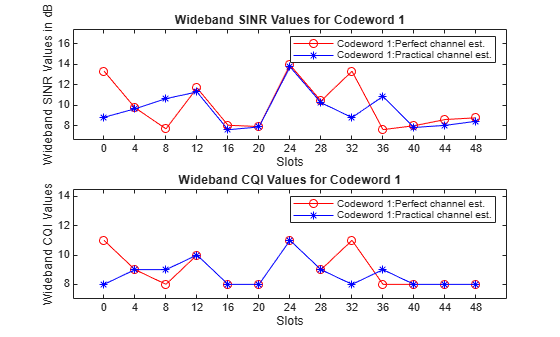Plot the subband SINR values and subband CQI values for each codeword for practical and perfect channel estimation scenarios. The plot shows the variations of SINR and CQI values across the subbands for a specified slot number (0-based) if the CQI is reported in that slot. Otherwise, plot is not generated because the CQI is not reported.

The `plotSubbandCQIAndSINR` function plots the CQI values across all subbands only when `CQIMode` is configured as `'Subband'`.

```% Provide slot number for which subband CQI values and PMI i2 indices must be plotted slotNumForSBType1 = 0; % Consider slot number as 0 (0-based) here, because CSI is % reported in that slot for the configured CSI-RS resource(s) plotSubbandCQIAndSINR(subbandCQIPractical,subbandCQIPerfect, ... SINRPerSubbandPerCWPractical,SINRPerSubbandPerCWPerfect,activeSlotNum,slotNumForSBType1);```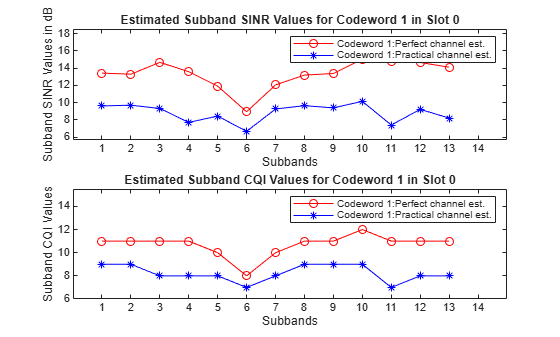#### Plot PMI and RI Indices

First plot in this section shows the variations of RI indices due to channel fading conditions, for practical and perfect channel estimation scenarios. The plot shows only the slots in which the CSI-RS is transmitted or in which RI is calculated.

#### Codebook Type `'Type1SinglePanel'`

The below code shows the variations of PMI due to channel fading conditions, for practical and perfect channel estimation scenarios.

The PMI indices are ${\mathit{i}}_{1}:\left[{\mathit{i}}_{1,1},{\mathit{i}}_{1,2},{\mathit{i}}_{1,3}\right]$ and ${\mathit{i}}_{2}$, as defined in TS 38.214 Section 5.2.2.2.1.

The first plot shows the rank and the corresponding PMI ${\mathit{i}}_{1}$ indices variation across multiple slots.

The second plot shows the variation in ${\mathit{i}}_{2}$ indices across the:

• Slots when the `PMIMode` is specified as `'Wideband'`

• Subbands in the specified slot when the `PMIMode` is specified as `'Subband'`

#### Codebook Type `'Type1MultiPanel'`

The below code shows the variations of PMI due to channel fading conditions, for practical and perfect channel estimation scenarios.

The PMI indices are ${\mathit{i}}_{1}:\left[{\mathit{i}}_{1,1},{\mathit{i}}_{1,2},{\mathit{i}}_{1,3},{\mathit{i}}_{1,4,1},{\mathit{i}}_{1,4,2},{\mathit{i}}_{1,4,3}\right]$ and ${\mathit{i}}_{2}:\left[{\mathit{i}}_{2,0},{\mathit{i}}_{2,1},{\mathit{i}}_{2,2}\right]$, as defined in TS 38.214 Section 5.2.2.2.2.

In this case, the first two plots show the rank and the corresponding PMI ${\mathit{i}}_{1}$ indices (${\mathit{i}}_{1,1}$ to ${\mathit{i}}_{1,4,3}$) variation across the slots.

The third plot shows the variation in each of the ${\mathit{i}}_{2}$ indices (${\mathit{i}}_{2,0}$ to ${\mathit{i}}_{2,2}$) across the:

• Slots when the `PMIMode` is specified as `'Wideband'`

• Subbands in the specified slot when the `PMIMode` is specified as `'Subband'`

#### Codebook Type `'Type2'` or `'eType2'`

The below code shows the grid of beams by highlighting the DFT vectors or beams that are used to construct the precoding matrix, for practical and perfect channel estimation scenarios. The plot shows the type II or enhanced type II PMI related information for a specified slot number (0-based), if PMI is reported in that slot. Otherwise, plot is not generated because the PMI is not reported.

For type II codebooks, the PMI indices are defined in TS 38.214 Section 5.2.2.2.3. These indices are:

• ${\mathit{i}}_{1}:\left[{\mathit{i}}_{1,1},{\mathit{i}}_{1,2},{\mathit{i}}_{1,3,1},{\mathit{i}}_{1,4,1}\right]$ and ${\mathit{i}}_{2}:\left[{\mathit{i}}_{2,1,1}\right]$ for single layer and `SubbandAmplitude` as `'false'`

• ${\mathit{i}}_{1}:\left[{\mathit{i}}_{1,1},{\mathit{i}}_{1,2},{\mathit{i}}_{1,3,1},{\mathit{i}}_{1,4,1}\right]$ and ${\mathit{i}}_{2}:\left[{\mathit{i}}_{2,1,1},{\mathit{i}}_{2,2,1}\right]$ for single layer and `SubbandAmplitude` as `'true'`

• ${\mathit{i}}_{1}:\left[{\mathit{i}}_{1,1},{\mathit{i}}_{1,2},{\mathit{i}}_{1,3,1},{\mathit{i}}_{1,4,1},{\mathit{i}}_{1,3,2},{\mathit{i}}_{1,4,2}\right]$ and ${\mathit{i}}_{2}:\left[{\mathit{i}}_{2,1,1},{\mathit{i}}_{2,1,2}\right]$ for two layers and `SubbandAmplitude` as `'false'`

• ${\mathit{i}}_{1}:\left[{\mathit{i}}_{1,1},{\mathit{i}}_{1,2},{\mathit{i}}_{1,3,1},{\mathit{i}}_{1,4,1},{\mathit{i}}_{1,3,2},{\mathit{i}}_{1,4,2}\right]$ and ${\mathit{i}}_{2}:\left[{\mathit{i}}_{2,1,1},{\mathit{i}}_{2,2,1},{\mathit{i}}_{2,1,2},{\mathit{i}}_{2,2,2}\right]$ for two layers and `SubbandAmplitude` as `'true'`

For enhanced type II codebooks, the PMI indices are defined in TS 38.214 Section 5.2.2.2.5. These indices are:

• ${\mathit{i}}_{1}:\left[{\mathit{i}}_{1,1},{\mathit{i}}_{1,2},{\mathit{i}}_{1,5},{\mathit{i}}_{1,6,1},{\mathit{i}}_{1,7,1},{\mathit{i}}_{1,8,1}\right]$ and ${\mathit{i}}_{2}:\left[{\mathit{i}}_{2,3,1},{\mathit{i}}_{2,4,1},{\mathit{i}}_{2,5,1}\right]$ for single layer

• ${\mathit{i}}_{1}:\left[{\mathit{i}}_{1,1},{\mathit{i}}_{1,2},{\mathit{i}}_{1,5},{\mathit{i}}_{1,6,1},{\mathit{i}}_{1,7,1},{\mathit{i}}_{1,8,1},{\mathit{i}}_{1,6,2},{\mathit{i}}_{1,7,2},{\mathit{i}}_{1,8,2}\right]$ and ${\mathit{i}}_{2}:\left[{\mathit{i}}_{2,3,1},{\mathit{i}}_{2,4,1},{\mathit{i}}_{2,5,1},{\mathit{i}}_{2,3,2},{\mathit{i}}_{2,4,2},{\mathit{i}}_{2,5,2}\right]$ for two layers

• ${\mathit{i}}_{1}:\left[{\mathit{i}}_{1,1},{\mathit{i}}_{1,2},{\mathit{i}}_{1,5},{\mathit{i}}_{1,6,1},{\mathit{i}}_{1,7,1},{\mathit{i}}_{1,8,1},{\mathit{i}}_{1,6,2},{\mathit{i}}_{1,7,2},{\mathit{i}}_{1,8,2},{\mathit{i}}_{1,6,3},{\mathit{i}}_{1,7,3},{\mathit{i}}_{1,8,3}\right]$ and ${\mathit{i}}_{2}:\left[{\mathit{i}}_{2,3,1},{\mathit{i}}_{2,4,1},{\mathit{i}}_{2,5,1},{\mathit{i}}_{2,3,2},{\mathit{i}}_{2,4,2},{\mathit{i}}_{2,5,2},{\mathit{i}}_{2,3,3},{\mathit{i}}_{2,4,3},{\mathit{i}}_{2,5,3}\right]$ for three layers

• ${\mathit{i}}_{1}:\left[{\mathit{i}}_{1,1},{\mathit{i}}_{1,2},{\mathit{i}}_{1,5},{\mathit{i}}_{1,6,1},{\mathit{i}}_{1,7,1},{\mathit{i}}_{1,8,1},{\mathit{i}}_{1,6,2},{\mathit{i}}_{1,7,2},{\mathit{i}}_{1,8,2},{\mathit{i}}_{1,6,3},{\mathit{i}}_{1,7,3},{\mathit{i}}_{1,8,3},{\mathit{i}}_{1,6,4},{\mathit{i}}_{1,7,4},{\mathit{i}}_{1,8,4}\right]$ and ${\mathit{i}}_{2}:\left[{\mathit{i}}_{2,3,1},{\mathit{i}}_{2,4,1},{\mathit{i}}_{2,5,1},{\mathit{i}}_{2,3,2},{\mathit{i}}_{2,4,2},{\mathit{i}}_{2,5,2},{\mathit{i}}_{2,3,3},{\mathit{i}}_{2,4,3},{\mathit{i}}_{2,5,3},{\mathit{i}}_{2,3,4},{\mathit{i}}_{2,4,4},{\mathit{i}}_{2,5,4}\right]$ for four layers

The first plot shows the rank variations, and second plot shows the grid of beams for practical and perfect channel estimation scenarios.

```% To see the PMI and RI plots for the type I single or multi-panel % codebooks, set the showType1PMIandRIPlots flag to true showType1PMIandRIPlots = true; % To see the PMI and RI plots for the type II codebooks, set the % showType2PMI flag to true showType2PMIRI = true; if (strcmpi(reportConfig.CodebookType,'Type1SinglePanel') || strcmpi(reportConfig.CodebookType,'Type1MultiPanel')) && showType1PMIandRIPlots plotType1PMIAndRI(pmiPracticalPerSlot,pmiPerfectPerSlot,riPracticalPerSlot, ... riPerfectPerSlot,activeSlotNum,slotNumForSBType1); elseif (strcmpi(reportConfig.CodebookType,'Type2') || strcmpi(reportConfig.CodebookType,'eType2')) && showType2PMIRI slotNumForType2PMI = 0; % 0-based plotType2PMIAndRI(pmiPracticalPerSlot,pmiPerfectPerSlot,riPracticalPerSlot, ... riPerfectPerSlot,reportConfig.PanelDimensions,reportConfig.NumberOfBeams,activeSlotNum,slotNumForType2PMI); end```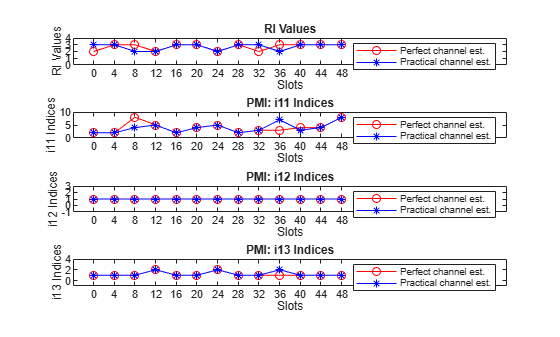### Summary and Further Exploration

This example shows how to compute downlink CSI parameters such as the CQI and PMI from type I single-panel codebooks and RI for a MIMO scenario with a TDL channel. The example also supports the computation of CSI parameters using type I multi-panel, type II, and enhanced type II codebooks.

You can modify carrier, channel, CSI-RS resource configurations, and CSI reporting configuration parameters (such as the codebook type, the mode of CQI and PMI reporting, and the subband size) and observe the variations in the computed CQI, PMI, and RI values across time (slots) and frequency (subbands).

### References

 3GPP TS 38.214. "NR; Physical layer procedures for data." 3rd Generation Partnership Project; Technical Specification Group Radio Access Network.

### Local Functions

This example uses these local functions to validate the CSI-RS configuration object and to plot the computed CQI, PMI, and RI values.

```function validateCSIRSConfig(carrier,csirs,nTxAnts) % Validates the CSI-RS configuration, given the carrier specific % configuration object, CSI-RS configuration object, and the number of % transmit antennas. % Validate the number of CSI-RS ports if ~isscalar(unique(csirs.NumCSIRSPorts)) error('nr5g:InvalidCSIRSPorts', ... 'All the CSI-RS resources must be configured to have the same number of CSI-RS ports.'); end % Validate the CDM lengths if ~iscell(csirs.CDMType) cdmType = {csirs.CDMType}; else cdmType = csirs.CDMType; end if (~all(strcmpi(cdmType,cdmType{1}))) error('nr5g:InvalidCSIRSCDMTypes', ... 'All the CSI-RS resources must be configured to have the same CDM lengths.'); end if nTxAnts ~= csirs.NumCSIRSPorts(1) error('nr5g:InvalidNumTxAnts',['Number of transmit antennas (' num2str(nTxAnts) ... ') must be equal to the number of CSI-RS ports (' num2str(csirs.NumCSIRSPorts(1)) ').']); end % Check for the overlap between the CSI-RS indices csirsInd = nrCSIRSIndices(carrier,csirs,"OutputResourceFormat",'cell'); numRes = numel(csirsInd); csirsIndAll = cell(1,numRes); ratioVal = csirs.NumCSIRSPorts(1)/prod(getCDMLengths(csirs)); for resIdx = 1:numRes if ~isempty(csirsInd{resIdx}) grid = nrResourceGrid(carrier,csirs.NumCSIRSPorts(1)); [~,tempInd] = nrExtractResources(csirsInd{resIdx},grid); if numel(tempInd)/numel(csirsInd{resIdx}) ~= ratioVal error('nr5g:OverlappedCSIRSREsSingleResource',['CSI-RS indices of resource ' ... num2str(resIdx) ' must be unique. Try changing the symbol or subcarrier locations.']); end csirsIndAll{resIdx} = tempInd(:); for idx = 1:resIdx-1 overlappedInd = ismember(csirsIndAll{idx},csirsIndAll{resIdx}); if any(overlappedInd) error('nr5g:OverlappedCSIRSREsMultipleResources',['The resource elements of the ' ... 'configured CSI-RS resources must not overlap. Try changing the symbol or ' ... 'subcarrier locations of CSI-RS resource ' num2str(idx) ' and resource ' num2str(resIdx) '.']); end end end end end function cdmLengths = getCDMLengths(csirs) % Returns the CDM lengths, given the CSI-RS configuration object. CDMType = csirs.CDMType; if ~iscell(csirs.CDMType) CDMType = {csirs.CDMType}; end CDMTypeOpts = {'noCDM','fd-CDM2','CDM4','CDM8'}; CDMLengthOpts = {[1 1],[2 1],[2 2],[2 4]}; cdmLengths = CDMLengthOpts{strcmpi(CDMTypeOpts,CDMType{1})}; end function [cqiPracticalPerSlot,subbandCQIPractical,pmiPracticalPerSlot,SINRPerSubbandPerCWPractical,cqiPerfectPerSlot, ... subbandCQIPerfect,pmiPerfectPerSlot,SINRPerSubbandPerCWPerfect,riPracticalPerSlot,riPerfectPerSlot] = fillInactiveSlots(cqiPracticalPerSlot, ... subbandCQIPractical,pmiPracticalPerSlot,SINRPerSubbandPerCWPractical,cqiPerfectPerSlot,subbandCQIPerfect,pmiPerfectPerSlot, ... SINRPerSubbandPerCWPerfect,riPracticalPerSlot,riPerfectPerSlot,reportConfig,totSlotsBinaryVec,activeSlots) % Returns the CQI, PMI, and RI related variables filled with NaNs in the % slots where NZP-CSI-RS is not present according to the codebook type from % the report configuration structure. Note that the CQI, PMI, and RI % variables are returned as empty if there are no NZP-CSI-RS resources, % that is, no active slots in the entire simulation duration. % Compute the indices of the slots and the number of slots in which % NZP-CSI-RS is not present inactiveSlotIdx = ~totSlotsBinaryVec; numInactiveSlots = nnz(inactiveSlotIdx); if ~isempty(activeSlots) numCQISBs = size(cqiPracticalPerSlot,1); % Get the codebook type codebookType = 'Type1SinglePanel'; if isfield(reportConfig,'CodebookType') codebookType = validatestring(reportConfig.CodebookType,{'Type1SinglePanel','Type1MultiPanel','Type2','eType2'},'fillInactiveSlots','CodebookType field'); end % Fill the CQI, PMI, and RI variables with NaNs in the slots where NZP-CSI-RS is % not present cqiPracticalPerSlot(:,:,inactiveSlotIdx) = NaN(numCQISBs,2,numInactiveSlots); subbandCQIPractical(:,:,inactiveSlotIdx) = NaN(numCQISBs,2,numInactiveSlots); SINRPerSubbandPerCWPractical(:,:,inactiveSlotIdx) = NaN(numCQISBs,2,numInactiveSlots); cqiPerfectPerSlot(:,:,inactiveSlotIdx) = NaN(numCQISBs,2,numInactiveSlots); subbandCQIPerfect(:,:,inactiveSlotIdx) = NaN(numCQISBs,2,numInactiveSlots); SINRPerSubbandPerCWPerfect(:,:,inactiveSlotIdx) = NaN(numCQISBs,2,numInactiveSlots); riPracticalPerSlot(inactiveSlotIdx) = NaN; riPerfectPerSlot(inactiveSlotIdx) = NaN; numi1Indices = 3; numi2Indices = 1; if strcmpi(codebookType,'Type1MultiPanel') numi1Indices = 6; numi2Indices = 3; end numPMISBs = size(pmiPerfectPerSlot(activeSlots(1)).i2,2); [pmiPerfectPerSlot(inactiveSlotIdx),pmiPracticalPerSlot(inactiveSlotIdx)] = deal(struct('i1',NaN(1,numi1Indices),'i2',NaN(numi2Indices,numPMISBs))); end end function plotWidebandCQIAndSINR(cqiPracticalPerSlot,cqiPerfectPerSlot,SINRPerSubbandPerCWPractical,SINRPerSubbandPerCWPerfect,activeSlotNum) % Plots the wideband SINR and wideband CQI values for each codeword % across all specified active slots (1-based) (in which the CQI is % reported as other than NaN) for practical and perfect channel % estimation cases. % Check if there are no slots in which NZP-CSI-RS is present if isempty(activeSlotNum) disp('No CQI data to plot, because there are no slots in which NZP-CSI-RS is present.'); return; end cqiPracticalPerCW = permute(cqiPracticalPerSlot(1,:,:),[1 3 2]); cqiPerfectPerCW = permute(cqiPerfectPerSlot(1,:,:),[1 3 2]); SINRPerCWPractical = permute(SINRPerSubbandPerCWPractical(1,:,:),[1 3 2]); SINRPerCWPerfect = permute(SINRPerSubbandPerCWPerfect(1,:,:),[1 3 2]); % Extract wideband CQI indices for slots where NZP-CSI-RS is present cqiPracticalPerCWActiveSlots = cqiPracticalPerCW(1,activeSlotNum,:); cqiPerfectPerCWActiveSlots = cqiPerfectPerCW(1,activeSlotNum,:); widebandSINRPractical = 10*log10(SINRPerCWPractical(1,activeSlotNum,:)); widebandSINRPerfect = 10*log10(SINRPerCWPerfect(1,activeSlotNum,:)); if isempty(reshape(cqiPracticalPerCWActiveSlots(:,:,1),1,[])) disp('No CQI data to plot, because all CQI values are NaNs.'); return; end figure(); plotWBCQISINR(widebandSINRPerfect,widebandSINRPractical,211,activeSlotNum,'SINR'); plotWBCQISINR(cqiPerfectPerCWActiveSlots,cqiPracticalPerCWActiveSlots,212,activeSlotNum,'CQI'); end function plotWBCQISINR(perfectVals,practicalVals,subplotIdx,activeSlotNum,inpText) % Plots the wideband SINR and wideband CQI values for each codeword % across all specified active slots (1-based) (in which the CQI is % reported as other than NaN) for practical and perfect channel % estimation cases. subplot(subplotIdx) plot(perfectVals(:,:,1),'r-o'); hold on; plot(practicalVals(:,:,1),'b-*'); if ~all(isnan(perfectVals(:,:,2))) % Two codewords hold on; plot(perfectVals(:,:,2),'r:s'); hold on; plot(practicalVals(:,:,2),'b:d'); title(['Wideband ' inpText ' Values for Codeword 1&2']); legend({'Codeword 1:Perfect channel est.','Codeword 1:Practical channel est.','Codeword 2:Perfect channel est.','Codeword 2:Practical channel est.'}); else title(['Wideband ' inpText ' Values for Codeword 1']); legend({'Codeword 1:Perfect channel est.','Codeword 1:Practical channel est.'}); end xlabel('Slots'); if strcmpi(inpText,'SINR') units = ' in dB'; else units = ''; end ylabel(['Wideband ' inpText ' Values' units]); xticks(1:size(perfectVals,2)); xTickLables = num2cell(activeSlotNum(:)-1); xticklabels(xTickLables); [lowerBound,upperBound] = bounds([practicalVals(:);perfectVals(:)]); ylim([lowerBound-1 upperBound+3.5]); end function plotSubbandCQIAndSINR(subbandCQIPractical,subbandCQIPerfect,SINRPerCWPractical,SINRPerCWPerfect,activeSlotNum,nslot) % Plots the SINR and CQI values for each codeword across all the subbands % for practical and perfect channel estimation cases for the given slot % number (0-based) among all specified active slots (1-based). The % function does not plot the values if CQIMode is 'Wideband' or if the % CQI and SINR values are all NaNs in the given slot. % Check if there are no slots in which NZP-CSI-RS is present if isempty(activeSlotNum) disp('No CQI data to plot, because there are no slots in which NZP-CSI-RS is present.'); return; end numSubbands = size(subbandCQIPractical,1); if numSubbands > 1 && ~any(nslot+1 == activeSlotNum) % Check if the CQI values are reported in the specified slot disp(['For the specified slot (' num2str(nslot) '), CQI values are not reported. Please choose another slot number.']); return; end % Plot subband CQI values if numSubbands > 1 % Subband mode subbandCQIPerCWPractical = subbandCQIPractical(2:end,:,nslot+1); subbandCQIPerCWPerfect = subbandCQIPerfect(2:end,:,nslot+1); subbandSINRPerCWPractical = 10*log10(SINRPerCWPractical(2:end,:,nslot+1)); subbandSINRPerCWPerfect = 10*log10(SINRPerCWPerfect(2:end,:,nslot+1)); figure(); plotSBCQISINR(subbandSINRPerCWPerfect,subbandSINRPerCWPractical,numSubbands,211,nslot,'SINR') plotSBCQISINR(subbandCQIPerCWPerfect,subbandCQIPerCWPractical,numSubbands,212,nslot,'CQI'); end end function plotSBCQISINR(perfectVals,practicalVals,numSubbands,subplotIdx,nslot,inpText) % Plots the SINR and CQI values for each codeword across all the subbands % for practical and perfect channel estimation cases for the given slot % number (0-based). The function does not plot the values if CQIMode is % 'Wideband' or if the CQI and SINR values are all NaNs in the given % slot. subplot(subplotIdx) plot(perfectVals(:,1),'ro-'); hold on; plot(practicalVals(:,1),'b*-'); if ~all(isnan(perfectVals(:,2))) % Two codewords hold on; plot(perfectVals(:,2),'rs:'); hold on; plot(practicalVals(:,2),'bd:'); legend({'Codeword 1:Perfect channel est.','Codeword 1:Practical channel est.','Codeword 2:Perfect channel est.','Codeword 2:Practical channel est.'}); title(['Estimated Subband ' inpText ' Values for Codeword 1&2 in Slot ' num2str(nslot)]); else % Single codeword legend({'Codeword 1:Perfect channel est.','Codeword 1:Practical channel est.'}); title(['Estimated Subband ' inpText ' Values for Codeword 1 in Slot ' num2str(nslot)]); end if strcmpi(inpText,'SINR') units = ' in dB'; else units = ''; end xlabel('Subbands'); ylabel(['Subband ' inpText ' Values' units]); xticks(1:numSubbands); xTickLables = num2cell(1:numSubbands); xticklabels(xTickLables); xlim([0 numSubbands+1]); [lowerBound,upperBound] = bounds([perfectVals(:);practicalVals(:)]); ylim([lowerBound-1 upperBound+3.5]); end function plotType1PMIAndRI(pmiPracticalPerSlot,pmiPerfectPerSlot,riPracticalPerSlot,riPerfectPerSlot,activeSlotNum,nslot) % Plots the RI and PMI i1 indices across all specified active slots % (1-based), for practical and perfect channel estimation scenarios. The % function also plots the i2 indices of practical and perfect channel % estimation scenarios across all specified active slots when the PMI % mode is 'Wideband' or plots i2 indices across all the subbands for the % specified slot number (0-based) when the PMI mode is 'Subband'. % Check if there are no slots in which NZP-CSI-RS is present if isempty(activeSlotNum) disp('No PMI and RI data to plot, because there are no slots in which NZP-CSI-RS is present.'); return; end numi1Indices = numel(pmiPracticalPerSlot(activeSlotNum(1)).i1); if numi1Indices == 6 codebookType = 'Type1MultiPanel'; else codebookType = 'Type1SinglePanel'; end % Extract wideband PMI indices (i1 values) for slots where NZP-CSI-RS % is present i1PerfectValsActiveSlots = reshape([pmiPerfectPerSlot(activeSlotNum).i1],numi1Indices,[])'; i1PracticalValsActiveSlots = reshape([pmiPracticalPerSlot(activeSlotNum).i1],numi1Indices,[])'; if isempty(i1PerfectValsActiveSlots) disp('No PMI and RI data to plot, because all PMI and RI values are NaNs.'); return; end figure; % Plot RI plotRI(riPracticalPerSlot,riPerfectPerSlot,activeSlotNum,411); % Extract and plot i11 indices i11PerfectVals = i1PerfectValsActiveSlots(:,1); i11PracticalVals = i1PracticalValsActiveSlots(:,1); plotIxxIndices(i11PerfectVals,i11PracticalVals,activeSlotNum,412,'i11'); % Extract and plot i12 indices i12PerfectVals = i1PerfectValsActiveSlots(:,2); i12PracticalVals = i1PracticalValsActiveSlots(:,2); plotIxxIndices(i12PerfectVals,i12PracticalVals,activeSlotNum,413,'i12'); % Extract and plot i13 indices i13PerfectVals = i1PerfectValsActiveSlots(:,3); i13PracticalVals = i1PracticalValsActiveSlots(:,3); plotIxxIndices(i13PerfectVals,i13PracticalVals,activeSlotNum,414,'i13'); % Plot the i141, i142 and i143 indices in type I multi-panel case if strcmpi(codebookType,'Type1MultiPanel') figure() % Extract and plot i141 indices i141PerfectVals = i1PerfectValsActiveSlots(:,4); i141PracticalVals = i1PracticalValsActiveSlots(:,4); plotIxxIndices(i141PerfectVals,i141PracticalVals,activeSlotNum,311,'i141'); % Extract and plot i142 indices i142PerfectVals = i1PerfectValsActiveSlots(:,5); i142PracticalVals = i1PracticalValsActiveSlots(:,5); plotIxxIndices(i142PerfectVals,i142PracticalVals,activeSlotNum,312,'i142'); % Extract and plot i143 indices i143PerfectVals = i1PerfectValsActiveSlots(:,6); i143PracticalVals = i1PracticalValsActiveSlots(:,6); plotIxxIndices(i143PerfectVals,i143PracticalVals,activeSlotNum,313,'i143'); end % Get the number of subbands numSubbands = size(pmiPracticalPerSlot(activeSlotNum(1)).i2,2); % Get the number of i2 indices according to codebook type numi2Indices = 1; if strcmpi(codebookType,'Type1MultiPanel') numi2Indices = 3; end % Get number of active slots numActiveSlots = numel(activeSlotNum); % Extract i2 values i2PerfectVals = reshape([pmiPerfectPerSlot(activeSlotNum).i2],[numSubbands,numi2Indices,numActiveSlots]); % Of size numActiveSlots-by-numi2Indices-numSubbands i2PracticalVals = reshape([pmiPracticalPerSlot(activeSlotNum).i2],[numSubbands,numi2Indices,numActiveSlots]); % Of size numActiveSlots-by-numi2Indices-numSubbands % Plot i2 values if numSubbands == 1 % Wideband mode figure; % In type I single-panel case, there is only one i2 index. The % first column of i2PerfectVals and i2PracticalVals corresponds to % i2 index. In type I multi-panel case, the i2 values are a set of % three indices i20, i21, and i22. Each column of i2PerfectVals and % i2PracticalVals correspond to i20, i21, and i22 indices. Extract % and plot the respective index values if strcmpi(codebookType,'Type1SinglePanel') % Extract and plot i2 values in each slot i2PerfectVals = reshape(i2PerfectVals(:,1,:),[],numActiveSlots).'; i2PracticalVals = reshape(i2PracticalVals(:,1,:),[],numActiveSlots).'; plotIxxIndices(i2PerfectVals,i2PracticalVals,activeSlotNum,111,'i2'); else % Extract and plot i20 values in each slot i20PerfectVals = reshape(i2PerfectVals(:,1,:),[],numActiveSlots).'; i20PracticalVals = reshape(i2PracticalVals(:,1,:),[],numActiveSlots).'; plotIxxIndices(i20PerfectVals,i20PracticalVals,activeSlotNum,311,'i20'); % Extract and plot i21 values in each slot i21PerfectVals = reshape(i2PerfectVals(:,2,:),[],numActiveSlots).'; i21PracticalVals = reshape(i2PracticalVals(:,2,:),[],numActiveSlots).'; plotIxxIndices(i21PerfectVals,i21PracticalVals,activeSlotNum,312,'i21'); % Extract and plot i22 values in each slot i22PerfectVals = reshape(i2PerfectVals(:,3,:),[],numActiveSlots).'; i22PracticalVals = reshape(i2PracticalVals(:,3,:),[],numActiveSlots).'; plotIxxIndices(i22PerfectVals,i22PracticalVals,activeSlotNum,313,'i22'); end else % Subband mode if any(nslot+1 == activeSlotNum) % In subband mode, plot the PMI i2 indices corresponding to the % specified slot number figure; if strcmpi(codebookType,'Type1SinglePanel') % Extract and plot i2 values pmiSBi2Perfect = pmiPerfectPerSlot(nslot+1).i2(1,:); pmiSBi2Practical = pmiPracticalPerSlot(nslot+1).i2(1,:); plotI2xIndices_SB(pmiSBi2Perfect,pmiSBi2Practical,numSubbands,nslot,111,'i2'); else % Extract and plot i20 values pmiSBi20Perfect = pmiPerfectPerSlot(nslot+1).i2(1,:); pmiSBi20Practical = pmiPracticalPerSlot(nslot+1).i2(1,:); plotI2xIndices_SB(pmiSBi20Perfect,pmiSBi20Practical,numSubbands,nslot,311,'i20'); % Extract and plot i21 values pmiSBi21Perfect = pmiPerfectPerSlot(nslot+1).i2(2,:); pmiSBi21Practical = pmiPracticalPerSlot(nslot+1).i2(2,:); plotI2xIndices_SB(pmiSBi21Perfect,pmiSBi21Practical,numSubbands,nslot,312,'i21'); % Extract and plot i22 values pmiSBi22Perfect = pmiPerfectPerSlot(nslot+1).i2(3,:); pmiSBi22Practical = pmiPracticalPerSlot(nslot+1).i2(3,:); plotI2xIndices_SB(pmiSBi22Perfect,pmiSBi22Practical,numSubbands,nslot,313,'i22'); end else disp(['For the specified slot (' num2str(nslot) '), PMI i2 indices are not reported. Please choose another slot number.']) end end end function plotType2PMIAndRI(pmiPracticalPerSlot,pmiPerfectPerSlot,riPracticalPerSlot,riPerfectPerSlot,panelDims,numBeams,activeSlotNum,nslot) % Plots the grid of beams by highlighting the beams that are used for the % precoding matrix generation for the specified slot number (0-based), % for practical and perfect channel estimation scenarios. % Check if there are no slots in which NZP-CSI-RS is present if isempty(activeSlotNum) disp('No PMI and RI data to plot, because there are no slots in which NZP-CSI-RS is present.'); return; end plotRI(riPracticalPerSlot,riPerfectPerSlot,activeSlotNum,111); if ~any(nslot+1 == activeSlotNum) disp(['For the specified slot (' num2str(nslot) '), PMI values are not reported. Please choose another slot number.']); else pmiPractical = pmiPracticalPerSlot(nslot+1); pmiPerfect = pmiPerfectPerSlot(nslot+1); figure(); plotType2GridOfBeams(pmiPractical,panelDims,numBeams,'Practical Channel Estimation Scenario',1); hold on; plotType2GridOfBeams(pmiPerfect,panelDims,numBeams,'Perfect Channel Estimation Scenario',2); end end function plotRI(riPracticalPerSlot,riPerfectPerSlot,activeSlotNum,subplotIndex) % Plots the RI values across all specified active slots (1-based), for % practical and perfect channel estimation scenarios. % Get number of active slots numActiveSlots = numel(activeSlotNum); % Extract RI values for slots where NZP-CSI-RS is present RIPerfectValsActiveSlots = riPerfectPerSlot(activeSlotNum)'; RIPracticalValsActiveSlots = riPracticalPerSlot(activeSlotNum)'; if isempty(RIPerfectValsActiveSlots) disp('No RI data to plot, because all RI values are NaNs.'); return; end figure; subplot(subplotIndex); plot(RIPerfectValsActiveSlots,'r-o'); hold on; plot(RIPracticalValsActiveSlots,'b-*'); xlabel('Slots') ylabel('RI Values'); xticks(1:numActiveSlots); xTickLables = num2cell(activeSlotNum(:)-1); xticklabels(xTickLables); [~,upperBound] = bounds([RIPerfectValsActiveSlots; RIPracticalValsActiveSlots]); xlim([0 numActiveSlots+8]); ylim([0 upperBound+1]); yticks(0:upperBound+1); title('RI Values') legend({'Perfect channel est.','Practical channel est.'}); end function plotType2GridOfBeams(PMISet,panelDims,numBeams,chEstType,subplotNum) % Plots the grid of beams by highlighting the beams that are used for the % type II codebook based precoding matrix generation. N1 = panelDims(1); N2 = panelDims(2); % Get the oversampling factors O1 = 4; O2 = 1 + 3*(N2 ~= 1); % Extract q1, q2 values qSet = PMISet.i1(1:2); q1 = qSet(1)-1; q2 = qSet(2)-1; % Extract i12 value i12 = PMISet.i1(3); s = 0; % Find the n1, n2 values for all the beams, as defined in TS 38.214 % Section 5.2.2.2.3 n1_i12 = zeros(1,numBeams); n2_i12 = zeros(1,numBeams); for beamIdxI = 0:numBeams-1 i12minussVal = i12 - s; xValues = numBeams-1-beamIdxI:N1*N2-1-beamIdxI; CValues = zeros(numel(xValues),1); for xIdx = 1:numel(xValues) if xValues(xIdx) >= numBeams-beamIdxI CValues(xIdx) = nchoosek(xValues(xIdx),numBeams-beamIdxI); end end indices = i12minussVal >= CValues; maxIdx = find(indices,1,'last'); xValue = xValues(maxIdx); ei = CValues(maxIdx); s = s+ei; ni = N1*N2 - 1 - xValue; n1_i12(beamIdxI+1) = mod(ni,N1); n2_i12(beamIdxI+1) = (ni-n1_i12(beamIdxI+1))/N1; end m1 = O1*(0:N1-1) + q1; m2 = O2*(0:N2-1) + q2; % Calculate the indices of orthogonal basis set which corresponds to % the reported i12 value m1_LBeams = O1*(n1_i12) + q1; m2_LBeams = O2*(n2_i12) + q2; OrthogonalBeams = [repmat(m1,1,length(m2));reshape(repmat(m2,length(m1),1),1,[])]'; % Plot the grid of beams numCirlcesInRow = N1*O1; numCirlcesInCol = N2*O2; subplot(2,1,subplotNum); circleRadius = 1; for colIdx = 0:numCirlcesInCol-1 for rowIdx = 0:numCirlcesInRow-1 p = nsidedpoly(1000, 'Center', [2*rowIdx 2*colIdx], 'Radius', circleRadius); if any(prod(OrthogonalBeams == [rowIdx colIdx],2)) h2 = plot(p, 'FaceColor', 'w','EdgeColor','r','LineWidth',2.5); hold on; if any(prod([m1_LBeams' m2_LBeams'] == [rowIdx colIdx],2)) h3 = plot(p, 'FaceColor', 'g','LineStyle','-.'); end else h1 = plot(p, 'FaceColor', 'w'); end hold on; end end rowLength = 2*circleRadius*O1; colLength = 2*circleRadius*O2; for n2 = 0:N2-1 for n1 = 0:N1-1 x1 = -1*circleRadius + rowLength*n1; x2 = x1 + rowLength; y1 = -1*circleRadius + colLength*n2; y2 = y1 + colLength; x = [x1, x2, x2, x1, x1]; y = [y1, y1, y2, y2, y1]; plot(x, y, 'b-', 'LineWidth', 2); hold on; end end xlabel('N1O1 beams'); ylabel('N2O2 beams'); axis equal; set(gca,'xtick',[],'ytick',[]); legend([h1 h2 h3],{'Oversampled DFT beams',['Orthogonal basis set with [q1 q2] = [' num2str(q1) ' ' num2str(q2) ']'],'Selected beam group'},'Location','northeast'); title(['Grid of Beams or DFT Vectors for ' chEstType]); end function plotIxxIndices(ixxPerfectVals,ixxPracticalVals,activeSlotNum,subplotInp,pmiIdxType) % Plots i11, i12, i13 indices in case of type I single-panel codebooks % and plots i141, i142, and i143 in case of type I multi-panel codebooks. % Plot ixx values subplot(subplotInp) plot(ixxPerfectVals,'r-o'); hold on; plot(ixxPracticalVals,'b-*'); xlabel('Slots') ylabel([pmiIdxType ' Indices']); % Get number of active slots numActiveSlots = numel(activeSlotNum); xticks(1:numActiveSlots); xTickLables = num2cell(activeSlotNum(:)-1); xticklabels(xTickLables); [lowerBound,upperBound] = bounds([ixxPerfectVals; ixxPracticalVals]); xlim([0 numActiveSlots+8]); ylim([lowerBound-2 upperBound+2]); title(['PMI: ' pmiIdxType ' Indices']); legend({'Perfect channel est.','Practical channel est.'}); end function plotI2xIndices_SB(pmiSBi2Perfect,pmiSBi2Practical,numSubbands,nslot,subplotInp,pmiIdxType) % Plots i2 indices in case of type I single-panel codebooks and plots % i20, i21, and i22 in case of type I multi-panel codebooks. subplot(subplotInp) plot(pmiSBi2Perfect,'r-o'); hold on; plot(pmiSBi2Practical,'b-*'); title(['PMI: ' pmiIdxType ' Indices for All Subbands in Slot ' num2str(nslot)]); xlabel('Subbands') ylabel([pmiIdxType ' Indices']); xticks(1:numSubbands); xticklabels(num2cell(1:numSubbands)); [lowerBound,upperBound] = bounds([pmiSBi2Perfect pmiSBi2Practical]); yticks(lowerBound:upperBound); yticklabels(num2cell(lowerBound:upperBound)); xlim([0 numSubbands+1]) ylim([lowerBound-1 upperBound+1]); legend({'Perfect channel est.','Practical channel est.'}); end```